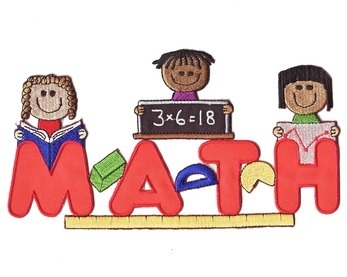# Math Vocabulary

•• ## STANDARDS FOR MATHEMATICAL PRACTICE THAT WE WORK ON CONTINUOUSLY THROUGHOUT THE YEAR ARE AS FOLLOWS:

1. Make sense of problems and persevere in solving them.
2. Reason abstractly and quantitatively.
3. Construct viable arguments and critique the reasoning of others.
4. Model with mathematics.
5. Use appropriate tools strategically.
6. Attend to precision.
7. Look for and make use of structure.
8. Look for- and express regularity in repeated reasoning.

• Chapter 1-Chapter 1 - Adding & Subtracting to Twenty

Chapter 1 Vocabulary

plus (+)
equals (=)
sum
make 10
doubles +1
doubles -1
subtract
minus (-)
difference
subtraction sentence
count up
fact family
part + part = whole
whole - part = part
doubles fact
related subtraction facts
fact family

Chapter 1 Concepts

Extend Facts to 20
Doubles Facts
Doubles +1; Doubles -1
Subtraction Concepts
Count Back to Subtract
Related Subtraction Facts
Count Up to Subtract
Make 10 to Subtract
Writing a Number Sentence
Fact Families
Use a Bar Model
Fact Patterns
Problem Solving Strategy: Choose the Operation
Problem Solving Strategy: Two-Step Problems
Problem-Solving Applications: Mixed Strategies

• ## Chapter 1 - Adding & Subtracting to Twenty

• Chapter 1 Vocabulary
plus (+)
equals (=)
sum
make 10
doubles +1
doubles -1
subtract
minus (-)
difference
subtraction sentence
count up
fact family
part + part = whole
whole - part = part
doubles fact
related subtraction facts
fact family
•
• Chapter 1 Concepts

Extend Facts to 20
Doubles Facts
Doubles +1; Doubles -1
Subtraction Concepts
Count Back to Subtract
Related Subtraction Facts